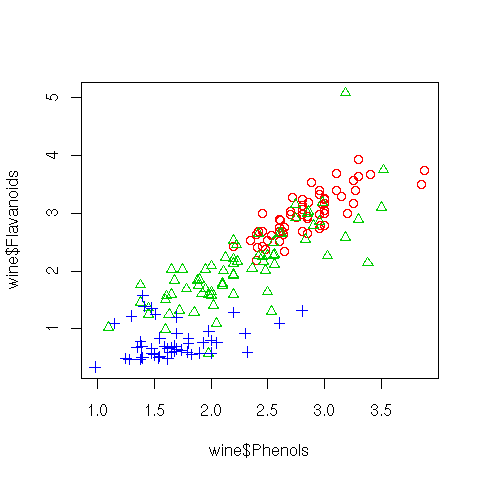DATA MINING
Desktop Survival Guide
by Graham Williams## Scatterplot

A scatterplot presents points in 2-dimensional space corresponding to a pair of chosen variables. R's plot function defaults to a scatterplot. Relationships between pairs of variables can be seen through the use of a scatterplot and clusters and outliers can begin to be identified.

Using the wine dataset a plot is created to display Phenols versus Flavanoids. To add a little more interest to the plot, a different symbol (and for colour devices, a different colour) is used to display the three different values of Type for each point. The symbols are set using Type as the argument to Roption[]pch, but after converting it to integers with as.integer. In a similar fashion, the colours are chosen to replace numbers in a transformation of the Type vector by indexing into the output of palette, achieved using lapply, and turning the result into a flat list, rather than a list of lists, using unlist.

We can start to understand that there is somewhat of a linear relationship between these two variables, and even more interesting is the clustering of Types.```iType <- as.integer(wine\$Type) colours <- unlist(lapply(iType, function(x){palette()[x+1]})) plot(wine\$Phenols, wine\$Flavanoids, col=colours, pch=iType) dev.off() ```

http://rattle.togaware.com/code/rplot-wine-scatter.R